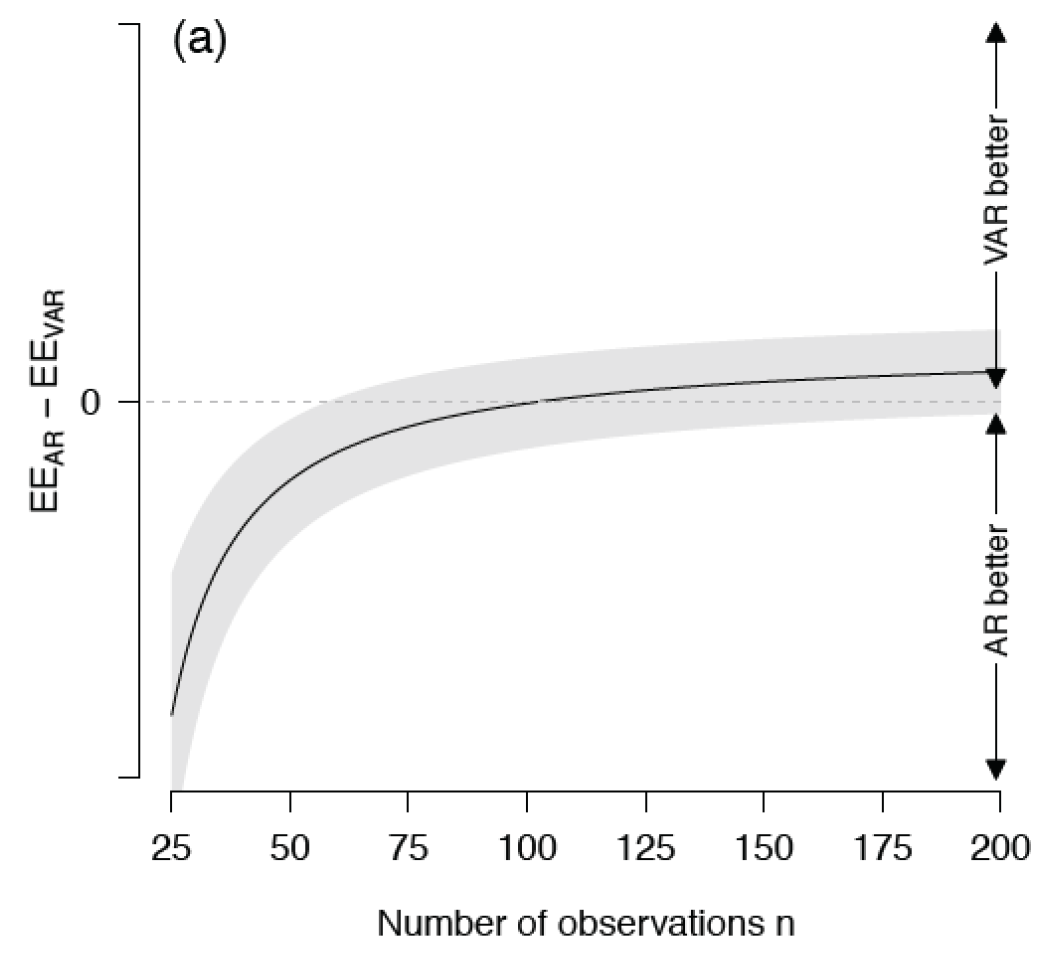22th February, 2019

## Context

• Most psychological theories are within-subject, but measurements are between-subject (Luce, 1997)
• There is no simple mapping between the two (Molenaar, 2004)

• Ubiquity of smartphones enables collecting intensive longitudinal data (Miller, 2012)
• Understanding humans as dynamical systems
• Goes in hand in hand with the network paradigm (Borsboom, 2017)## VAR Model

• We observe some realization of the (discrete-time) $$p$$-dimensional stochastic process

$\{Y(t): t \in T\}$

• with state space $$Y(t) \in \mathbb{R}^p$$
• Vector autoregressive (VAR) model assumes

$\mathbf{y}_t = \mathbf{\nu} + \mathbf{A}_1 \mathbf{y}_{t-1} + \ldots + \mathbf{A}_l \mathbf{y}_{t-1} + \mathbf{\epsilon}_t$

• $$\nu \in \mathbb{R}^p$$ is the intercept
• $$A_l \in \mathbb{R}^{p \times p}$$ describes the coefficients at lag $$l$$
• $$\mathbf{\epsilon}_t$$ are the stochastic innovations at time $$t$$, for which

\begin{align} \mathbf{\epsilon}_t \sim \mathcal{N}(0, \Sigma) \\[0.5em] \mathbb{E}[\mathbf{\epsilon}_t\mathbf{\epsilon}_{t-1}] = 0 \end{align}

• VAR process is covariance-stationary, i.e. its first and second moment are time-invariant

## AR Model

• Lag $$l = 1$$ VAR model requires estimation of $$A^{p \times p}$$
• This may lead to high variance of the estimates when data is poor
• Reduce variance by introducing bias: set all off-diagonal elements to zero
• This saves estimating $$p \times (p - 1)$$ parameters

$A_{\text{VAR}} = \begin{pmatrix} \alpha_{11} & \alpha_{12} & \alpha_{13} & \alpha_{14} & \alpha_{15} & \alpha_{16} \\ \alpha_{21} & \alpha_{22} & \alpha_{23} & \alpha_{24} & \alpha_{25} & \alpha_{26} \\ \alpha_{31} & \alpha_{32} & \alpha_{33} & \alpha_{34} & \alpha_{35} & \alpha_{36} \\ \alpha_{41} & \alpha_{42} & \alpha_{43} & \alpha_{44} & \alpha_{45} & \alpha_{46} \\ \alpha_{51} & \alpha_{52} & \alpha_{53} & \alpha_{54} & \alpha_{55} & \alpha_{56} \\ \alpha_{61} & \alpha_{62} & \alpha_{63} & \alpha_{64} & \alpha_{65} & \alpha_{66} \end{pmatrix}$

$A_{\text{AR}} = \begin{pmatrix} \alpha_{11} & 0 & 0 & 0 & 0 & 0 \\ 0 & \alpha_{22} & 0 & 0 & 0 & 0 \\ 0 & 0 & \alpha_{33} & 0 & 0 & 0 \\ 0 & 0 & 0 & \alpha_{44} & 0 & 0 \\ 0 & 0 & 0 & 0 & \alpha_{55} & 0 \\ 0 & 0 & 0 & 0 & 0 & \alpha_{66} \\ \end{pmatrix}$